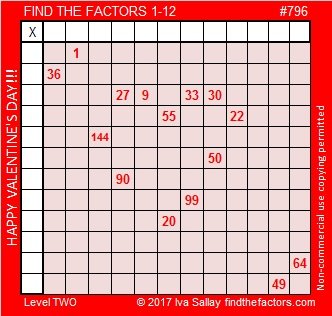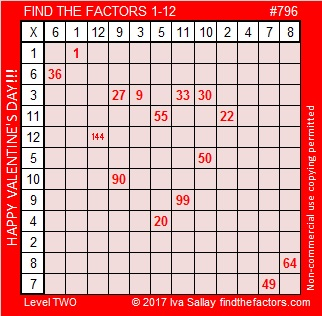# 796 and a Valentine

Here’s a puzzle you can print, cut out, and give as a valentine:You may know the divisibility rules for these powers of two:

• If the last digit of a number is divisible by 2, the whole number is divisible by 2.
• If the last two digits are divisible by 4, the whole number is divisible by 4.
• If the last three digits are divisible by 8, the whole number is divisible by 8.

But I’m going to apply some other time-saving but possibly more confusing divisibility rules to the number 796:

• 796 is divisible by 2 because 6 is an even number.
• 796 is divisible by 4 because even number 6 is NOT divisible by 4, and 9 is an odd number.
• 796 is NOT divisible by 8 because 96 is divisible by 8, and 7 is an odd number.

Because 796 is divisible by 4 but not by 8, it can be written as the sum of 8 consecutive numbers:

• 96 + 97 + 98 + 99 + 100 + 101 + 102 + 103 = 796

796 is also the sum of all the prime numbers from 113 to 149:

• 113 + 127 + 131 + 137 + 139 + 149 = 796

Here is the factoring information for 796:

• 796 is a composite number.
• Prime factorization: 796 = 2 x 2 x 199, which can be written 796 = (2^2) x 199
• The exponents in the prime factorization are 2 and 1. Adding one to each and multiplying we get (2 + 1)(1 + 1) = 3 x 2  = 6. Therefore 796 has exactly 6 factors.
• Factors of 796: 1, 2, 4, 199, 398, 796
• Factor pairs: 796 = 1 x 796, 2 x 398, or 4 x 199
• Taking the factor pair with the largest square number factor, we get √796 = (√4)(√199) = 2√199 ≈ 28.21347.This site uses Akismet to reduce spam. Learn how your comment data is processed.